Скачать презентацию Chapter 16 Arbitrage Restrictions on Option Prices

bb8cd3731fe2b7fdd5647cdaf45e9862.ppt

• Количество слайдов: 46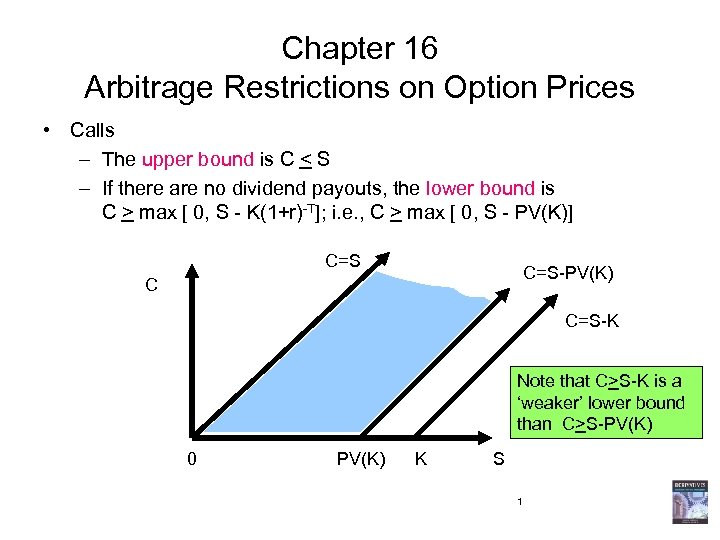Chapter 16 Arbitrage Restrictions on Option Prices • Calls – The upper bound is C < S – If there are no dividend payouts, the lower bound is C > max [ 0, S - K(1+r)-T]; i. e. , C > max [ 0, S - PV(K)] C=S-PV(K) C C=S-K Note that C>S-K is a ‘weaker’ lower bound than C>S-PV(K) 0 PV(K) K S 1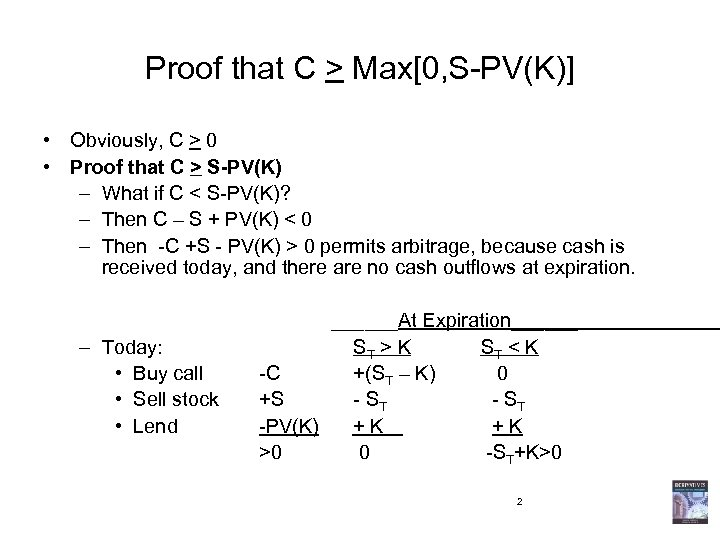Proof that C > Max[0, S-PV(K)] • Obviously, C > 0 • Proof that C > S-PV(K) – What if C < S-PV(K)? – Then C – S + PV(K) < 0 – Then -C +S - PV(K) > 0 permits arbitrage, because cash is received today, and there are no cash outflows at expiration. – Today: • Buy call • Sell stock • Lend ______At Expiration______ ST > K ST < K -C +(ST – K) 0 +S - ST -PV(K) + K >0 0 -ST+K>0 2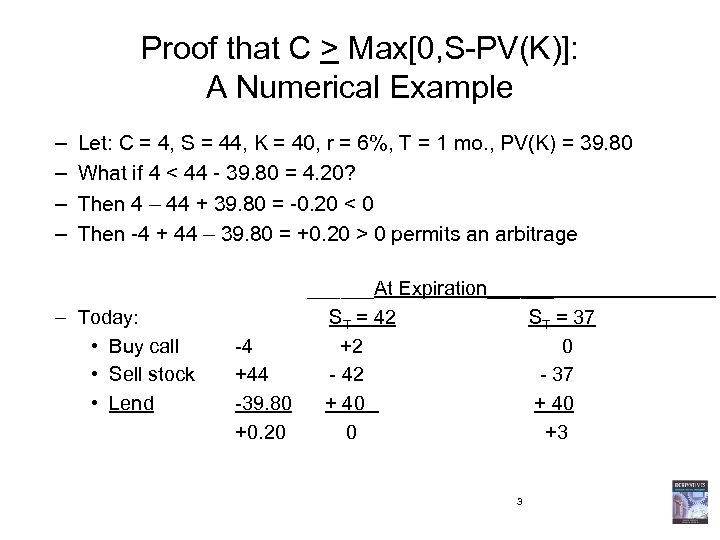Proof that C > Max[0, S-PV(K)]: A Numerical Example – – Let: C = 4, S = 44, K = 40, r = 6%, T = 1 mo. , PV(K) = 39. 80 What if 4 < 44 - 39. 80 = 4. 20? Then 4 – 44 + 39. 80 = -0. 20 < 0 Then -4 + 44 – 39. 80 = +0. 20 > 0 permits an arbitrage – Today: • Buy call • Sell stock • Lend ______At Expiration______ ST = 42 ST = 37 -4 +2 0 +44 - 42 - 37 -39. 80 + 40 +0. 20 0 +3 3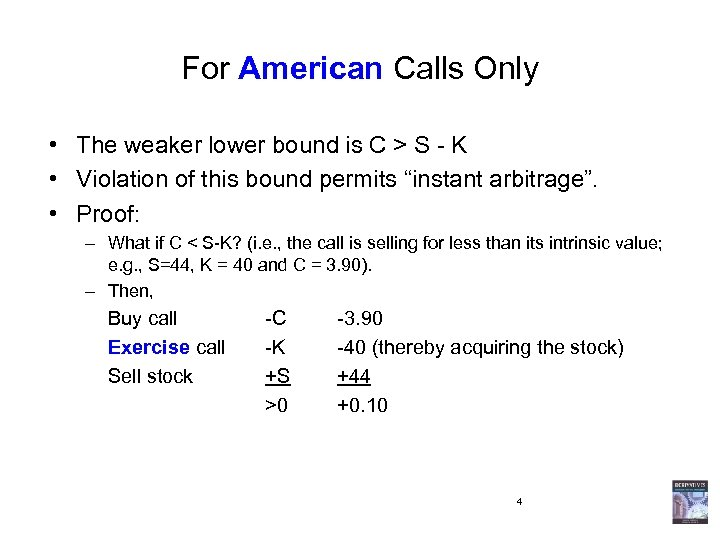For American Calls Only • The weaker lower bound is C > S - K • Violation of this bound permits “instant arbitrage”. • Proof: – What if C < S-K? (i. e. , the call is selling for less than its intrinsic value; e. g. , S=44, K = 40 and C = 3. 90). – Then, Buy call Exercise call Sell stock -C -K +S >0 -3. 90 -40 (thereby acquiring the stock) +44 +0. 10 4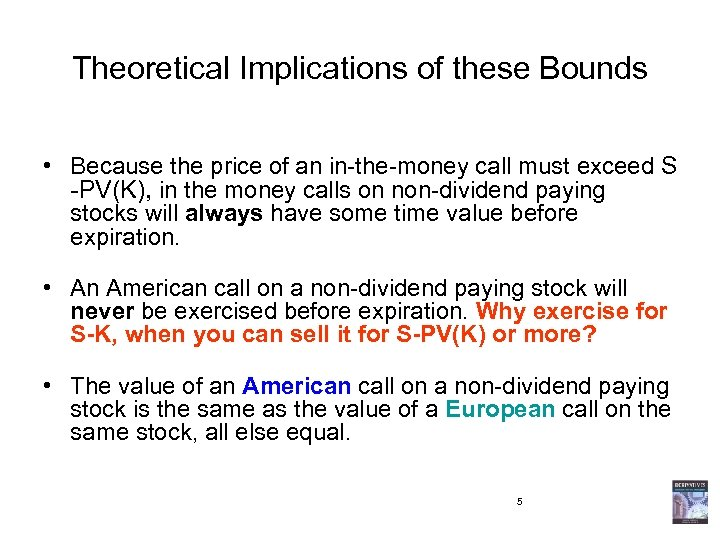Theoretical Implications of these Bounds • Because the price of an in-the-money call must exceed S -PV(K), in the money calls on non-dividend paying stocks will always have some time value before expiration. • An American call on a non-dividend paying stock will never be exercised before expiration. Why exercise for S-K, when you can sell it for S-PV(K) or more? • The value of an American call on a non-dividend paying stock is the same as the value of a European call on the same stock, all else equal. 5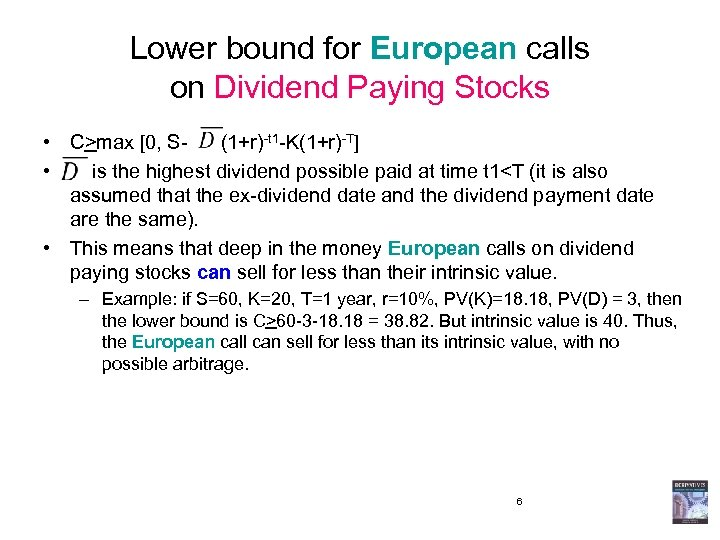Lower bound for European calls on Dividend Paying Stocks • C>max [0, S- (1+r)-t 1 -K(1+r)-T] • is the highest dividend possible paid at time t 160 -3 -18. 18 = 38. 82. But intrinsic value is 40. Thus, the European call can sell for less than its intrinsic value, with no possible arbitrage. 6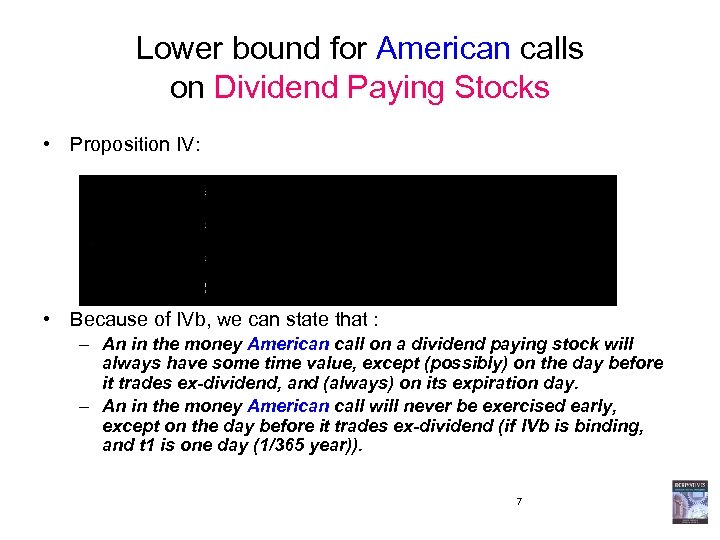Lower bound for American calls on Dividend Paying Stocks • Proposition IV: • Because of IVb, we can state that : – An in the money American call on a dividend paying stock will always have some time value, except (possibly) on the day before it trades ex-dividend, and (always) on its expiration day. – An in the money American call will never be exercised early, except on the day before it trades ex-dividend (if IVb is binding, and t 1 is one day (1/365 year)). 7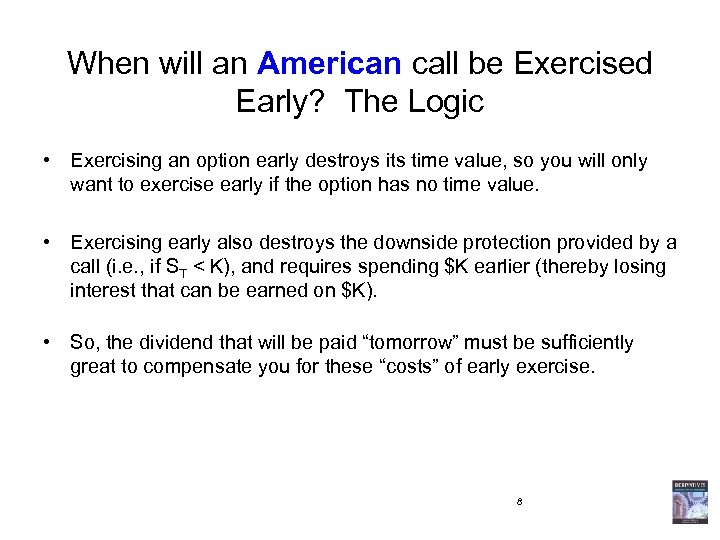When will an American call be Exercised Early? The Logic • Exercising an option early destroys its time value, so you will only want to exercise early if the option has no time value. • Exercising early also destroys the downside protection provided by a call (i. e. , if ST < K), and requires spending \$K earlier (thereby losing interest that can be earned on \$K). • So, the dividend that will be paid “tomorrow” must be sufficiently great to compensate you for these “costs” of early exercise. 8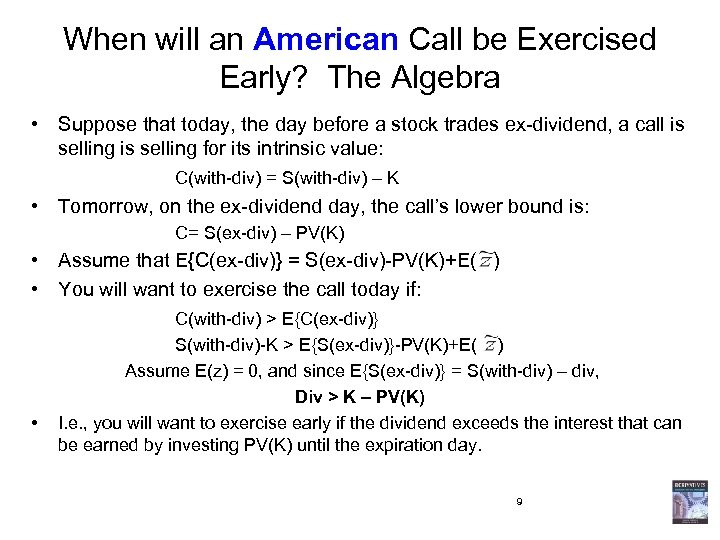When will an American Call be Exercised Early? The Algebra • Suppose that today, the day before a stock trades ex-dividend, a call is selling for its intrinsic value: C(with-div) = S(with-div) – K • Tomorrow, on the ex-dividend day, the call’s lower bound is: C= S(ex-div) – PV(K) • Assume that E{C(ex-div)} = S(ex-div)-PV(K)+E( ) • You will want to exercise the call today if: • C(with-div) > E{C(ex-div)} S(with-div)-K > E{S(ex-div)}-PV(K)+E( ) Assume E(z) = 0, and since E{S(ex-div)} = S(with-div) – div, Div > K – PV(K) I. e. , you will want to exercise early if the dividend exceeds the interest that can be earned by investing PV(K) until the expiration day. 9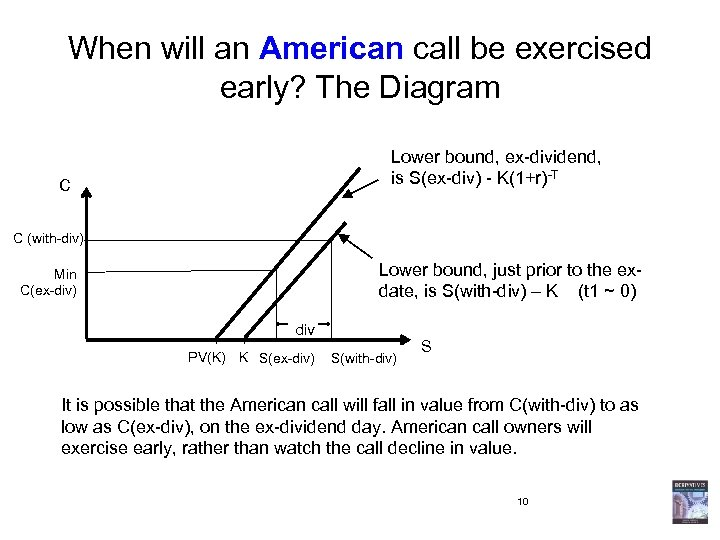When will an American call be exercised early? The Diagram Lower bound, ex-dividend, is S(ex-div) - K(1+r)-T C C (with-div) Lower bound, just prior to the exdate, is S(with-div) – K (t 1 ~ 0) Min C(ex-div) div PV(K) K S(ex-div) S(with-div) S It is possible that the American call will fall in value from C(with-div) to as low as C(ex-div), on the ex-dividend day. American call owners will exercise early, rather than watch the call decline in value. 10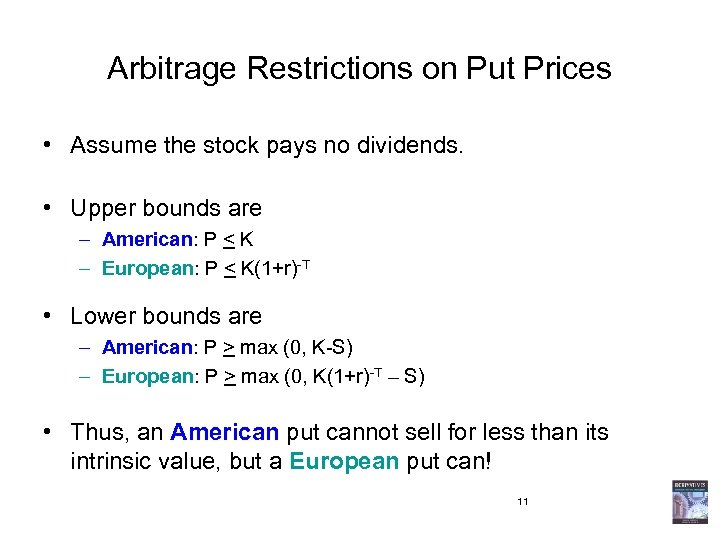Arbitrage Restrictions on Put Prices • Assume the stock pays no dividends. • Upper bounds are – American: P < K – European: P < K(1+r)-T • Lower bounds are – American: P > max (0, K-S) – European: P > max (0, K(1+r)-T – S) • Thus, an American put cannot sell for less than its intrinsic value, but a European put can! 11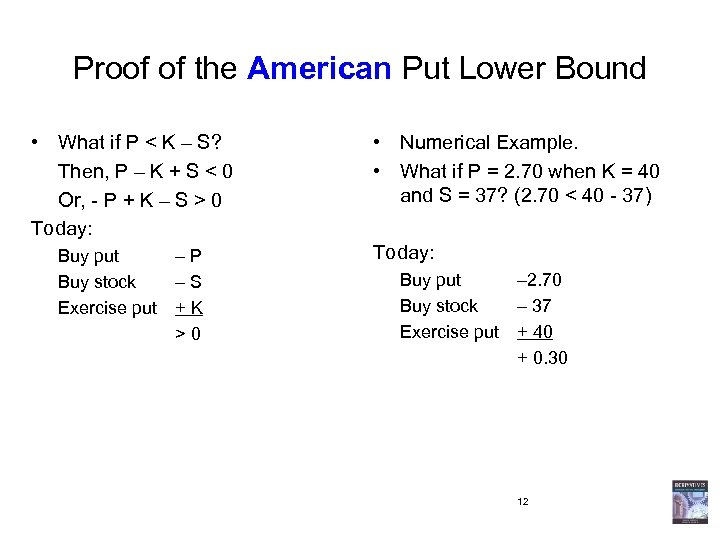Proof of the American Put Lower Bound • What if P < K – S? Then, P – K + S < 0 Or, - P + K – S > 0 Today: Buy put – P Buy stock – S Exercise put + K > 0 • Numerical Example. • What if P = 2. 70 when K = 40 and S = 37? (2. 70 < 40 - 37) Today: Buy put – 2. 70 Buy stock – 37 Exercise put + 40 + 0. 30 12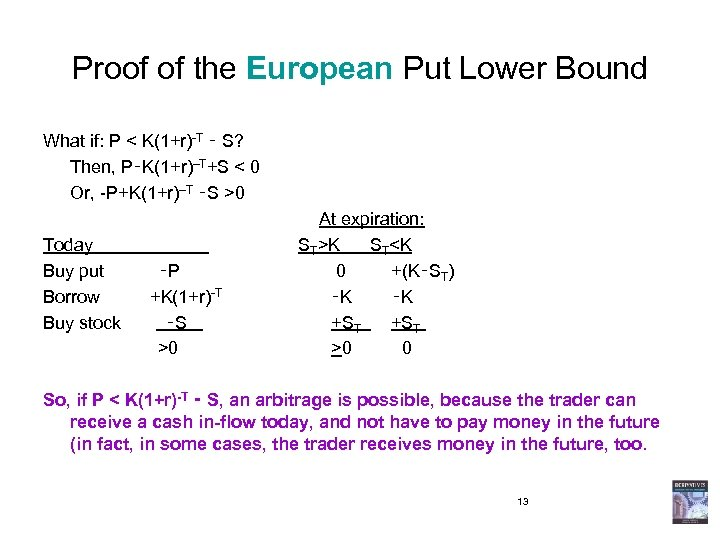Proof of the European Put Lower Bound What if: P < K(1+r)-T ‑ S? Then, P‑K(1+r)–T+S < 0 Or, -P+K(1+r)–T ‑S >0 At expiration: Today ST>K ST0 0 So, if P < K(1+r)-T ‑ S, an arbitrage is possible, because the trader can receive a cash in-flow today, and not have to pay money in the future (in fact, in some cases, the trader receives money in the future, too. 13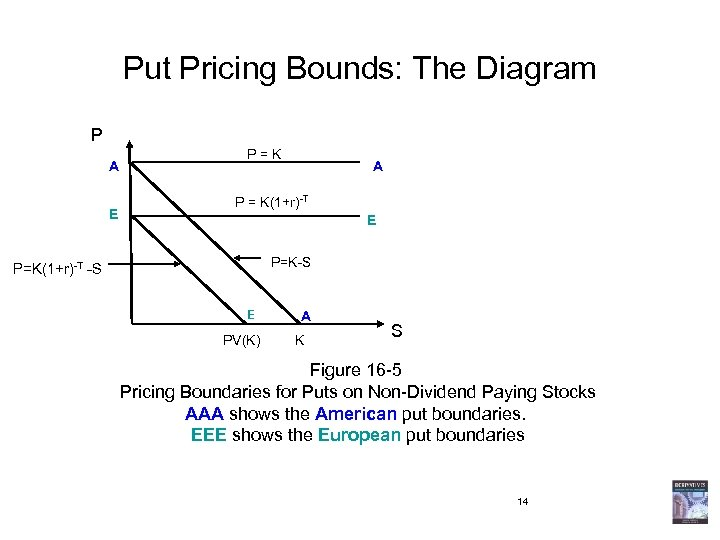Put Pricing Bounds: The Diagram P A E P = K A P = K(1+r)-T E P=K-S P=K(1+r)-T -S E PV(K) A K S Figure 16 -5 Pricing Boundaries for Puts on Non-Dividend Paying Stocks AAA shows the American put boundaries. EEE shows the European put boundaries 14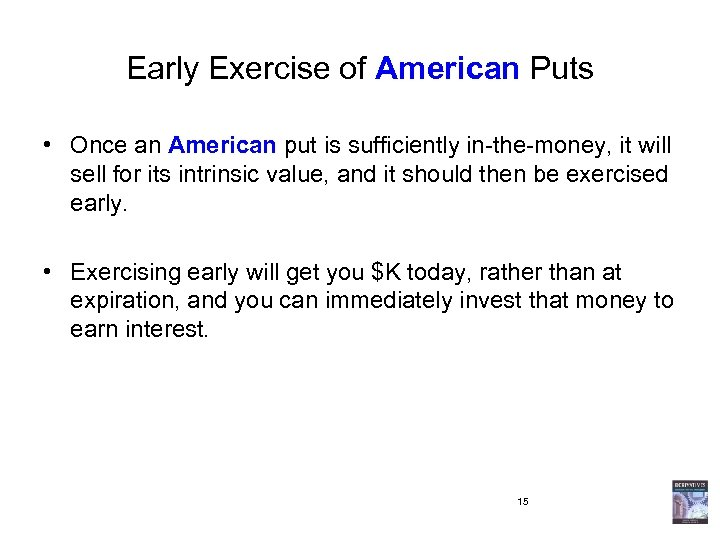Early Exercise of American Puts • Once an American put is sufficiently in-the-money, it will sell for its intrinsic value, and it should then be exercised early. • Exercising early will get you \$K today, rather than at expiration, and you can immediately invest that money to earn interest. 15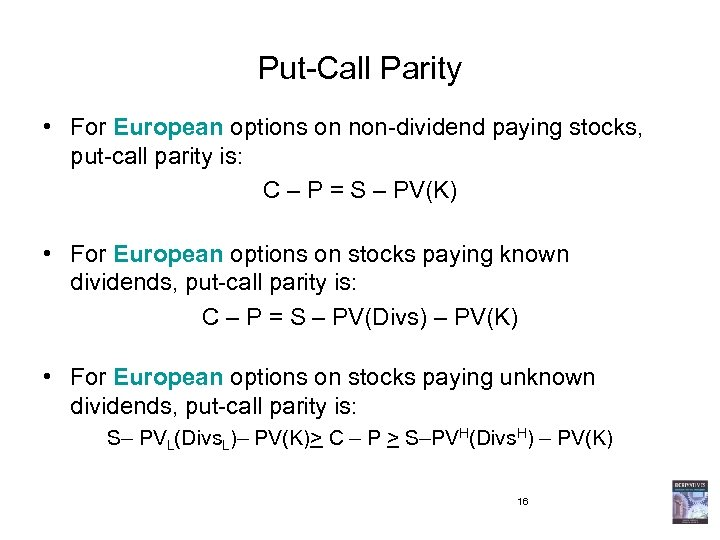Put-Call Parity • For European options on non-dividend paying stocks, put-call parity is: C – P = S – PV(K) • For European options on stocks paying known dividends, put-call parity is: C – P = S – PV(Divs) – PV(K) • For European options on stocks paying unknown dividends, put-call parity is: S– PVL(Divs. L)– PV(K)> C – P > S–PVH(Divs. H) – PV(K) 16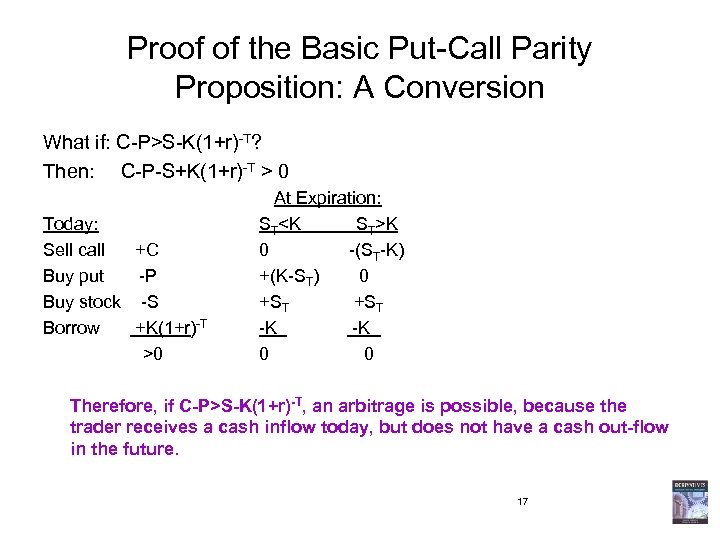Proof of the Basic Put-Call Parity Proposition: A Conversion What if: C-P>S-K(1+r)-T? Then: C-P-S+K(1+r)-T > 0 At Expiration: Today: STK Sell call +C 0 -(ST-K) Buy put -P +(K-ST) 0 Buy stock -S +ST Borrow +K(1+r)-T -K >0 0 Therefore, if C-P>S-K(1+r)-T, an arbitrage is possible, because the trader receives a cash inflow today, but does not have a cash out-flow in the future. 17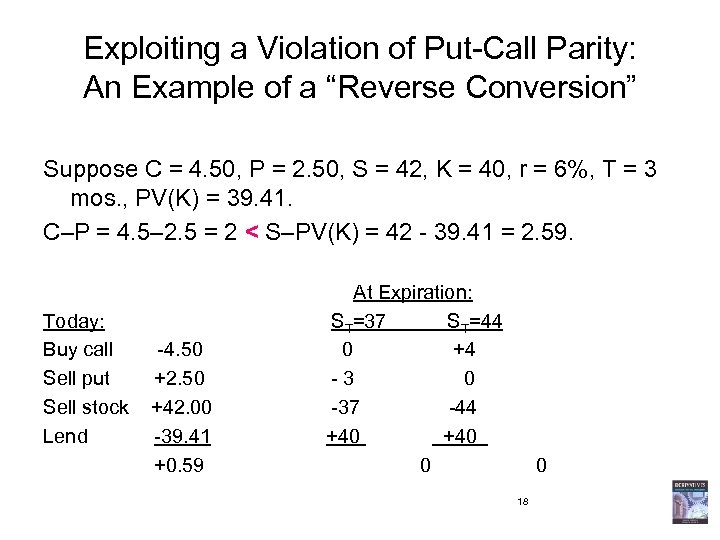Exploiting a Violation of Put-Call Parity: An Example of a “Reverse Conversion” Suppose C = 4. 50, P = 2. 50, S = 42, K = 40, r = 6%, T = 3 mos. , PV(K) = 39. 41. C–P = 4. 5– 2. 5 = 2 < S–PV(K) = 42 - 39. 41 = 2. 59. At Expiration: Today: ST=37 ST=44 Buy call -4. 50 +4 Sell put +2. 50 - 3 0 Sell stock +42. 00 -37 -44 Lend -39. 41 +40 +0. 59 0 18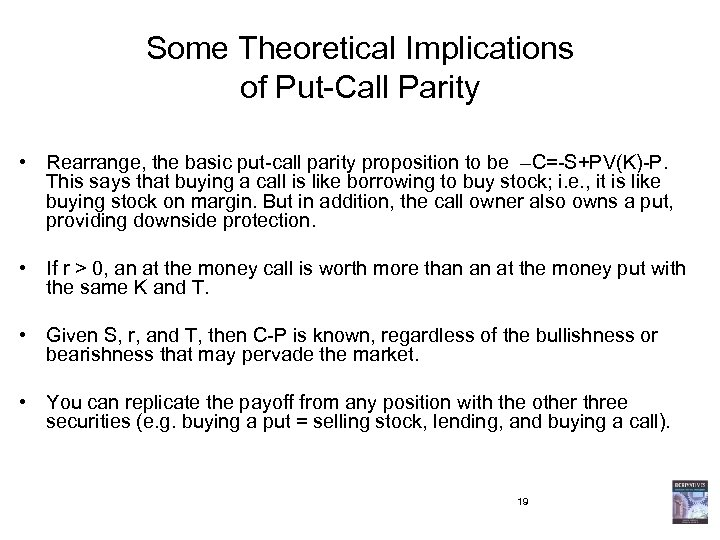Some Theoretical Implications of Put-Call Parity • Rearrange, the basic put-call parity proposition to be –C=-S+PV(K)-P. This says that buying a call is like borrowing to buy stock; i. e. , it is like buying stock on margin. But in addition, the call owner also owns a put, providing downside protection. • If r > 0, an at the money call is worth more than an at the money put with the same K and T. • Given S, r, and T, then C-P is known, regardless of the bullishness or bearishness that may pervade the market. • You can replicate the payoff from any position with the other three securities (e. g. buying a put = selling stock, lending, and buying a call). 19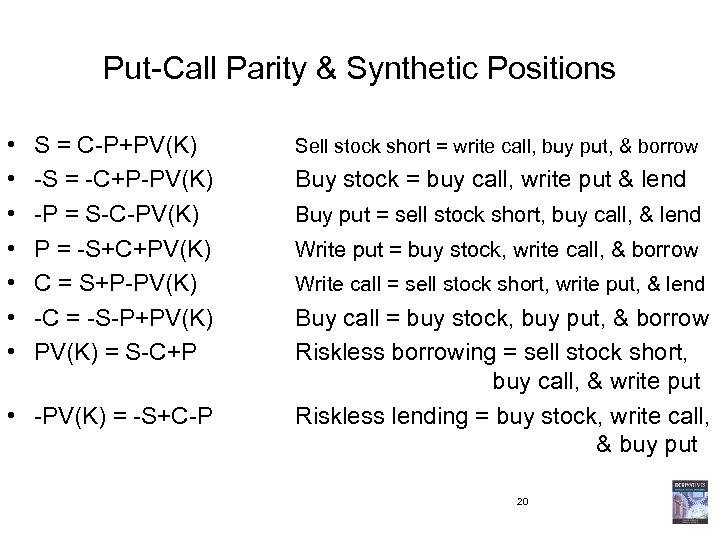Put-Call Parity & Synthetic Positions • • S = C-P+PV(K) -S = -C+P-PV(K) -P = S-C-PV(K) P = -S+C+PV(K) C = S+P-PV(K) -C = -S-P+PV(K) = S-C+P • -PV(K) = -S+C-P Sell stock short = write call, buy put, & borrow Buy stock = buy call, write put & lend Buy put = sell stock short, buy call, & lend Write put = buy stock, write call, & borrow Write call = sell stock short, write put, & lend Buy call = buy stock, buy put, & borrow Riskless borrowing = sell stock short, buy call, & write put Riskless lending = buy stock, write call, & buy put 20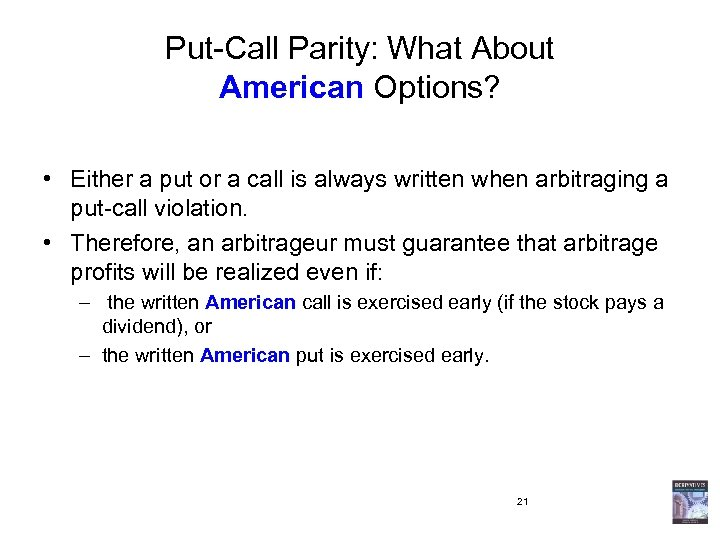Put-Call Parity: What About American Options? • Either a put or a call is always written when arbitraging a put-call violation. • Therefore, an arbitrageur must guarantee that arbitrage profits will be realized even if: – the written American call is exercised early (if the stock pays a dividend), or – the written American put is exercised early. 21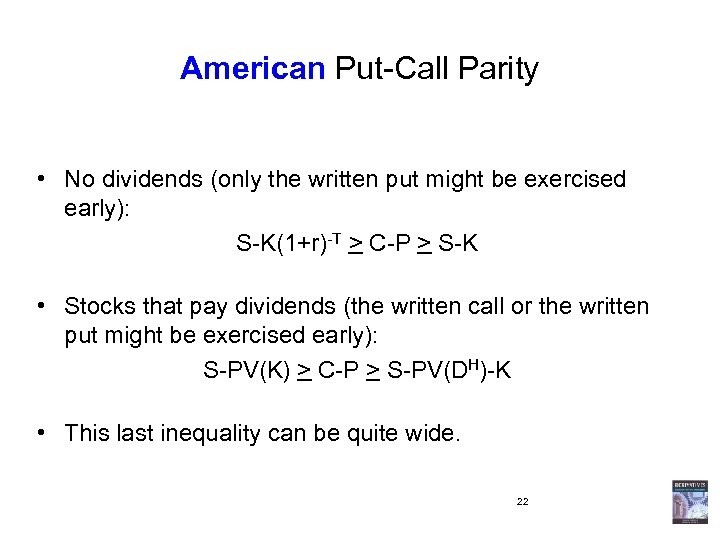American Put-Call Parity • No dividends (only the written put might be exercised early): S-K(1+r)-T > C-P > S-K • Stocks that pay dividends (the written call or the written put might be exercised early): S-PV(K) > C-P > S-PV(DH)-K • This last inequality can be quite wide. 22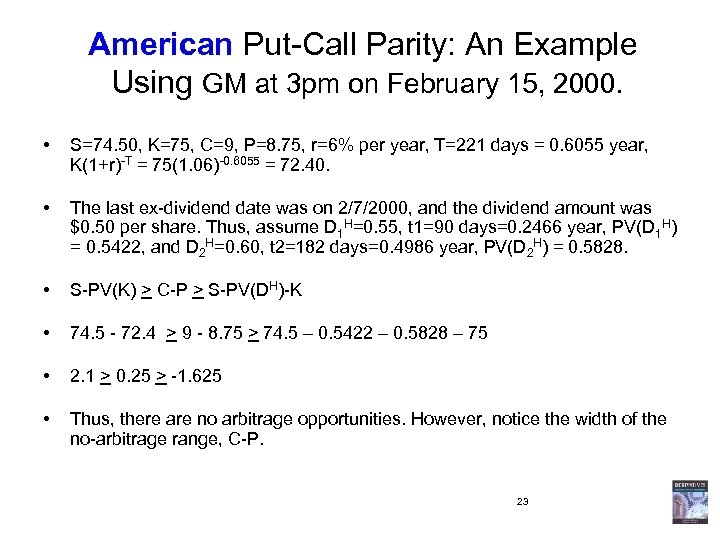American Put-Call Parity: An Example Using GM at 3 pm on February 15, 2000. • S=74. 50, K=75, C=9, P=8. 75, r=6% per year, T=221 days = 0. 6055 year, K(1+r)-T = 75(1. 06)-0. 6055 = 72. 40. • The last ex-dividend date was on 2/7/2000, and the dividend amount was \$0. 50 per share. Thus, assume D 1 H=0. 55, t 1=90 days=0. 2466 year, PV(D 1 H) = 0. 5422, and D 2 H=0. 60, t 2=182 days=0. 4986 year, PV(D 2 H) = 0. 5828. • S-PV(K) > C-P > S-PV(DH)-K • 74. 5 - 72. 4 > 9 - 8. 75 > 74. 5 – 0. 5422 – 0. 5828 – 75 • 2. 1 > 0. 25 > -1. 625 • Thus, there are no arbitrage opportunities. However, notice the width of the no-arbitrage range, C-P. 23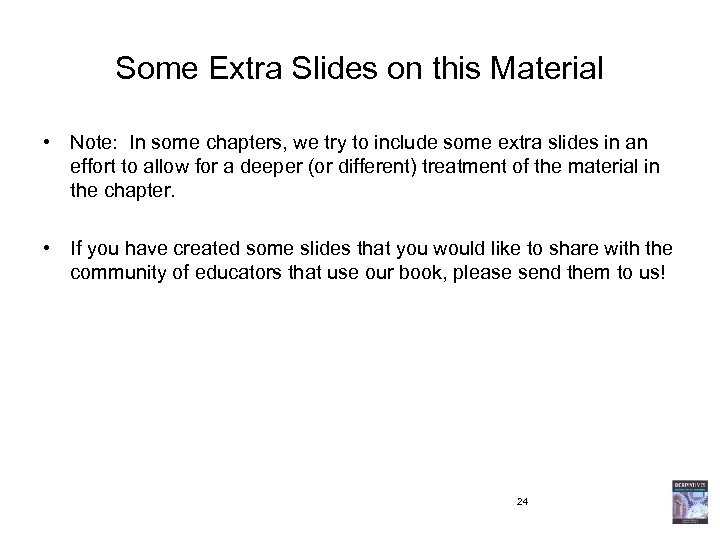Some Extra Slides on this Material • Note: In some chapters, we try to include some extra slides in an effort to allow for a deeper (or different) treatment of the material in the chapter. • If you have created some slides that you would like to share with the community of educators that use our book, please send them to us! 24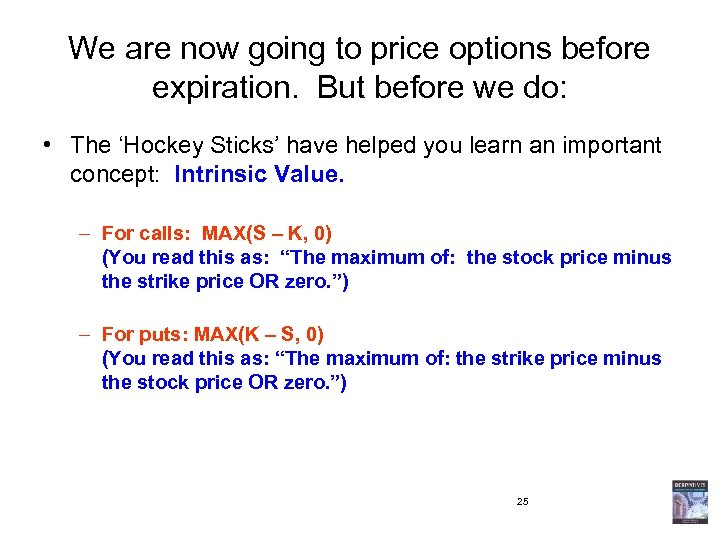We are now going to price options before expiration. But before we do: • The ‘Hockey Sticks’ have helped you learn an important concept: Intrinsic Value. – For calls: MAX(S – K, 0) (You read this as: “The maximum of: the stock price minus the strike price OR zero. ”) – For puts: MAX(K – S, 0) (You read this as: “The maximum of: the strike price minus the stock price OR zero. ”) 25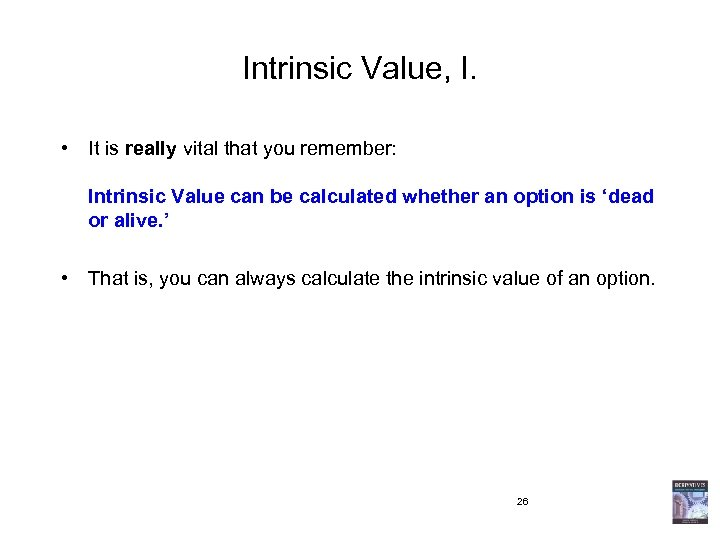Intrinsic Value, I. • It is really vital that you remember: Intrinsic Value can be calculated whether an option is ‘dead or alive. ’ • That is, you can always calculate the intrinsic value of an option. 26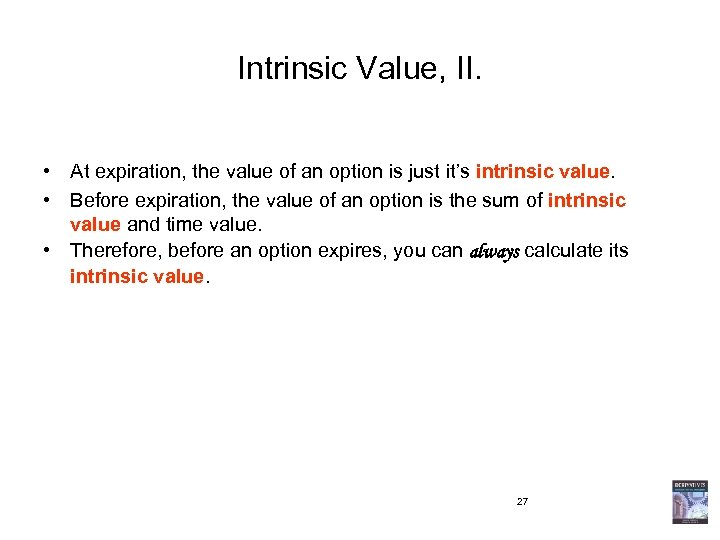Intrinsic Value, II. • At expiration, the value of an option is just it’s intrinsic value. • Before expiration, the value of an option is the sum of intrinsic value and time value. • Therefore, before an option expires, you can always calculate its intrinsic value. 27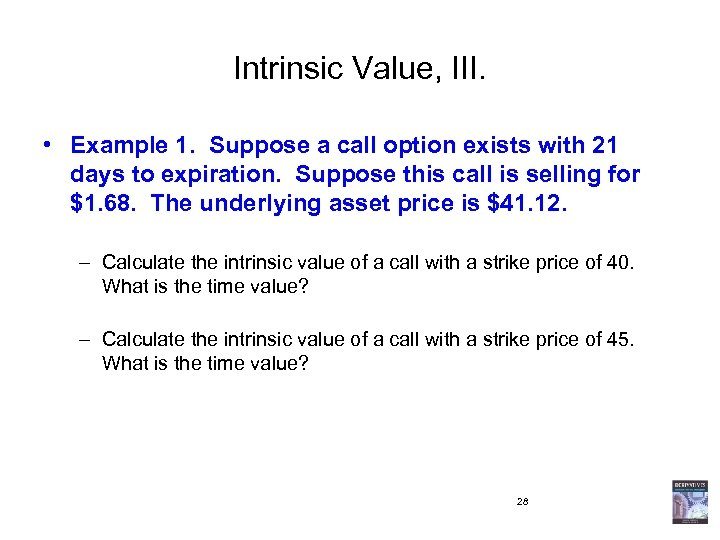Intrinsic Value, III. • Example 1. Suppose a call option exists with 21 days to expiration. Suppose this call is selling for \$1. 68. The underlying asset price is \$41. 12. – Calculate the intrinsic value of a call with a strike price of 40. What is the time value? – Calculate the intrinsic value of a call with a strike price of 45. What is the time value? 28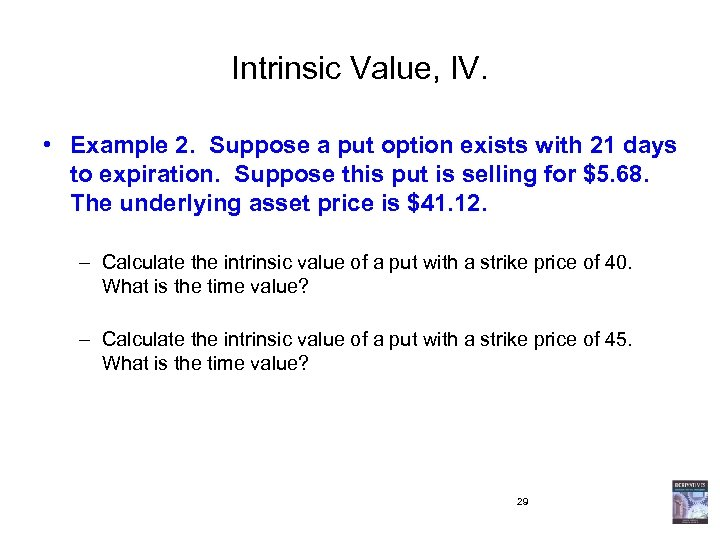Intrinsic Value, IV. • Example 2. Suppose a put option exists with 21 days to expiration. Suppose this put is selling for \$5. 68. The underlying asset price is \$41. 12. – Calculate the intrinsic value of a put with a strike price of 40. What is the time value? – Calculate the intrinsic value of a put with a strike price of 45. What is the time value? 29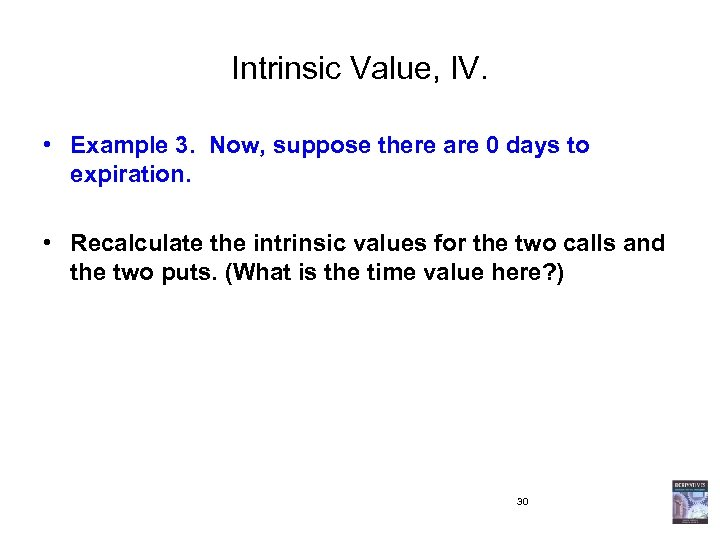Intrinsic Value, IV. • Example 3. Now, suppose there are 0 days to expiration. • Recalculate the intrinsic values for the two calls and the two puts. (What is the time value here? ) 30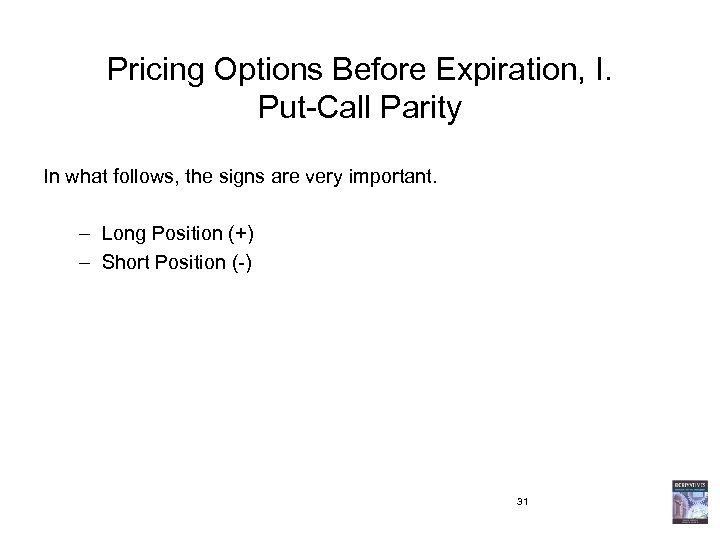Pricing Options Before Expiration, I. Put-Call Parity In what follows, the signs are very important. – Long Position (+) – Short Position (-) 31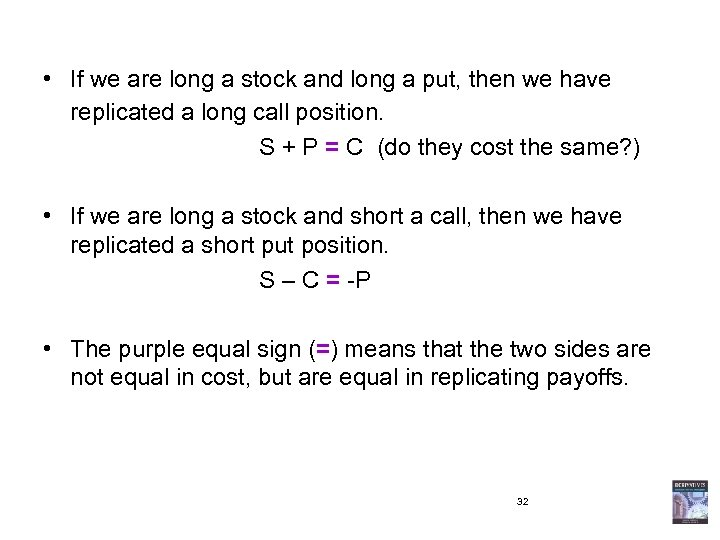• If we are long a stock and long a put, then we have replicated a long call position. S + P = C (do they cost the same? ) • If we are long a stock and short a call, then we have replicated a short put position. S – C = -P • The purple equal sign (=) means that the two sides are not equal in cost, but are equal in replicating payoffs. 32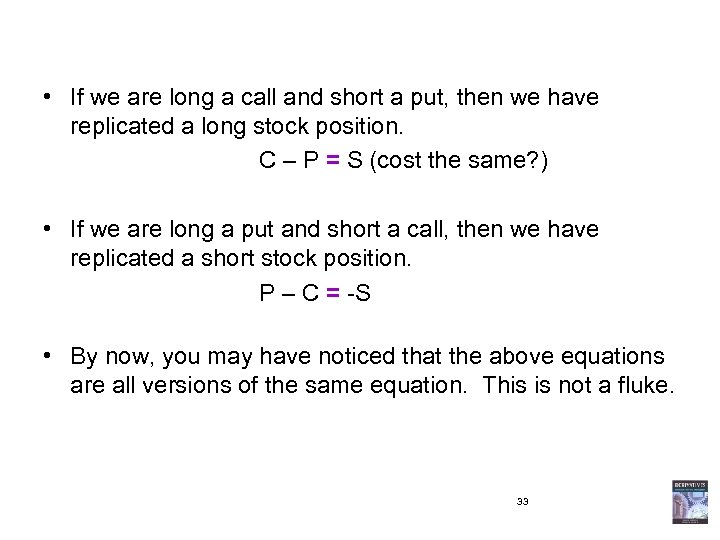• If we are long a call and short a put, then we have replicated a long stock position. C – P = S (cost the same? ) • If we are long a put and short a call, then we have replicated a short stock position. P – C = -S • By now, you may have noticed that the above equations are all versions of the same equation. This is not a fluke. 33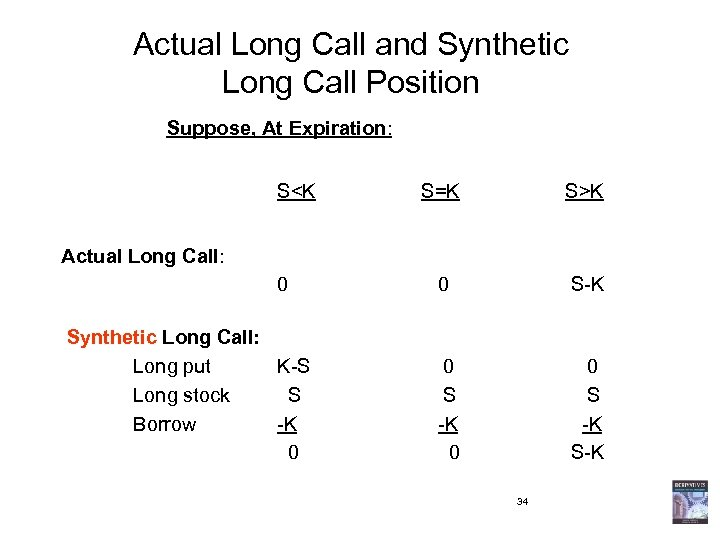Actual Long Call and Synthetic Long Call Position Suppose, At Expiration: SK 0 0 S-K 0 S -K 0 0 S -K S-K Actual Long Call: Synthetic Long Call: Long put K-S Long stock S Borrow -K 0 34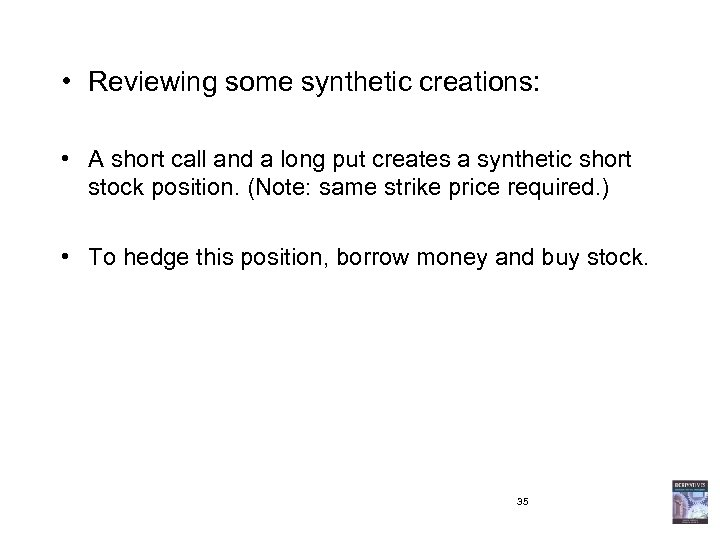• Reviewing some synthetic creations: • A short call and a long put creates a synthetic short stock position. (Note: same strike price required. ) • To hedge this position, borrow money and buy stock. 35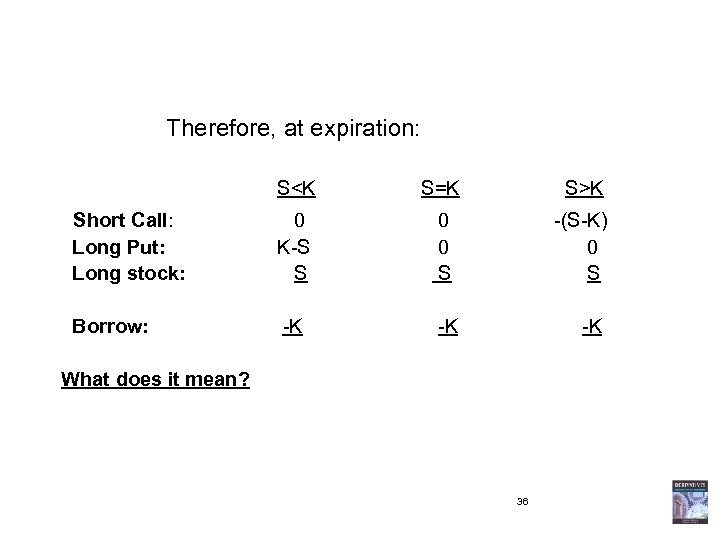Therefore, at expiration: SK -K -(S-K) 0 S -K What does it mean? 36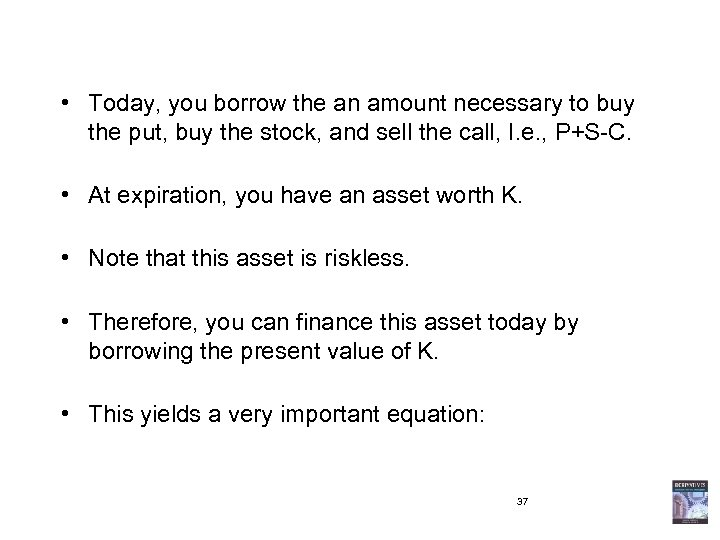• Today, you borrow the an amount necessary to buy the put, buy the stock, and sell the call, I. e. , P+S-C. • At expiration, you have an asset worth K. • Note that this asset is riskless. • Therefore, you can finance this asset today by borrowing the present value of K. • This yields a very important equation: 37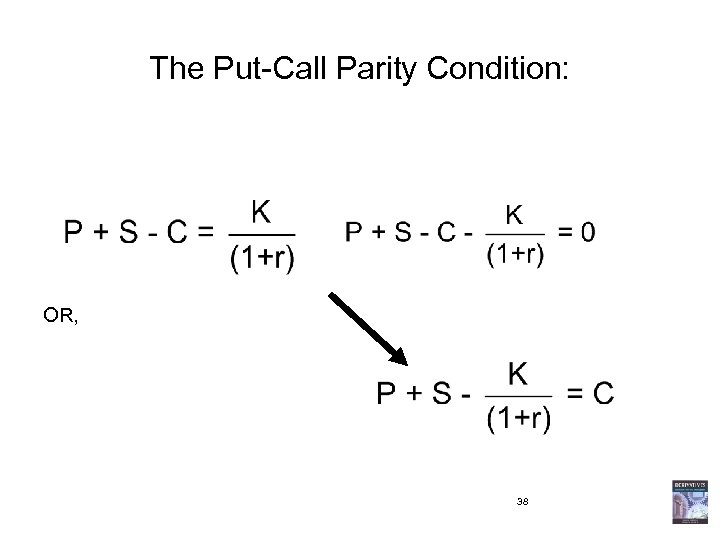The Put-Call Parity Condition: OR, 38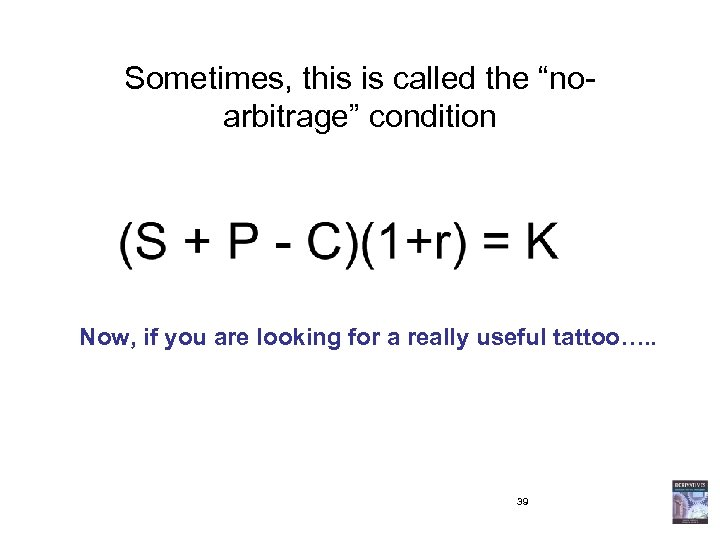Sometimes, this is called the “noarbitrage” condition Now, if you are looking for a really useful tattoo…. . 39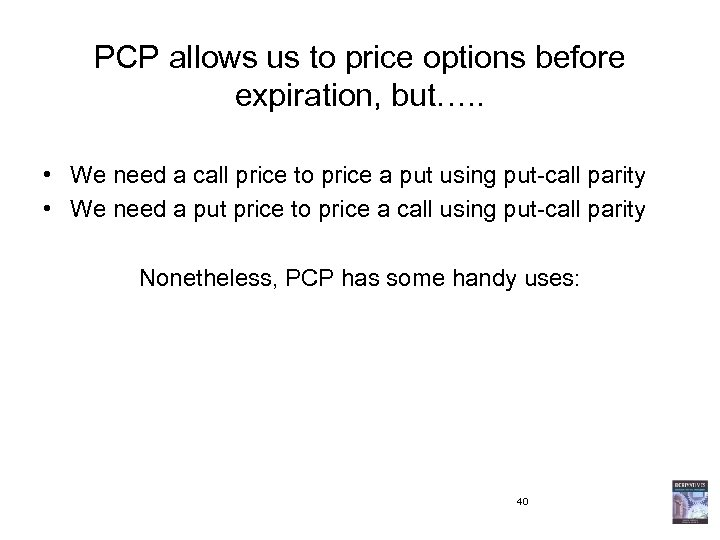PCP allows us to price options before expiration, but…. . • We need a call price to price a put using put-call parity • We need a put price to price a call using put-call parity Nonetheless, PCP has some handy uses: 40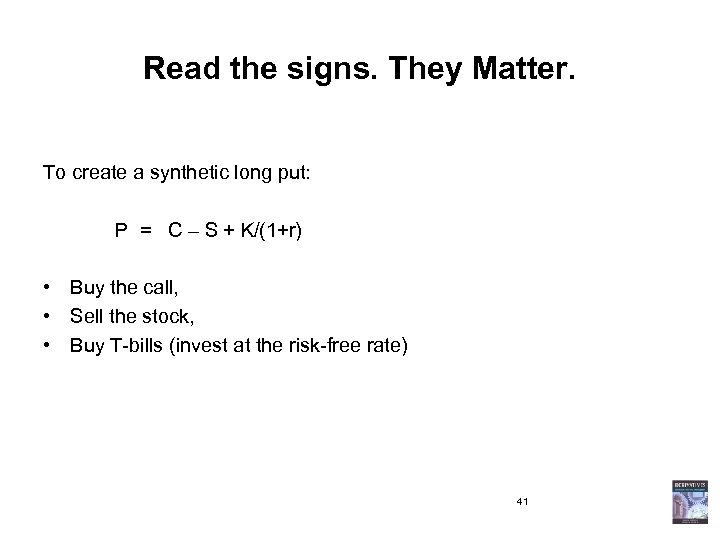Read the signs. They Matter. To create a synthetic long put: P = C – S + K/(1+r) • Buy the call, • Sell the stock, • Buy T-bills (invest at the risk-free rate) 41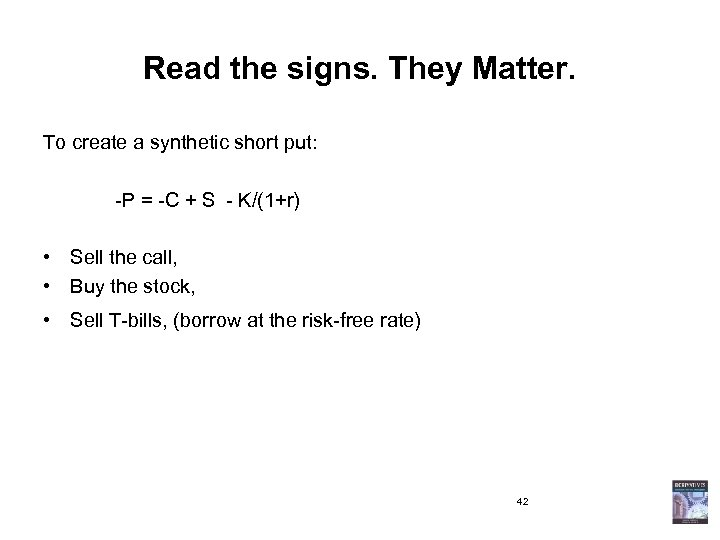Read the signs. They Matter. To create a synthetic short put: -P = -C + S - K/(1+r) • Sell the call, • Buy the stock, • Sell T-bills, (borrow at the risk-free rate) 42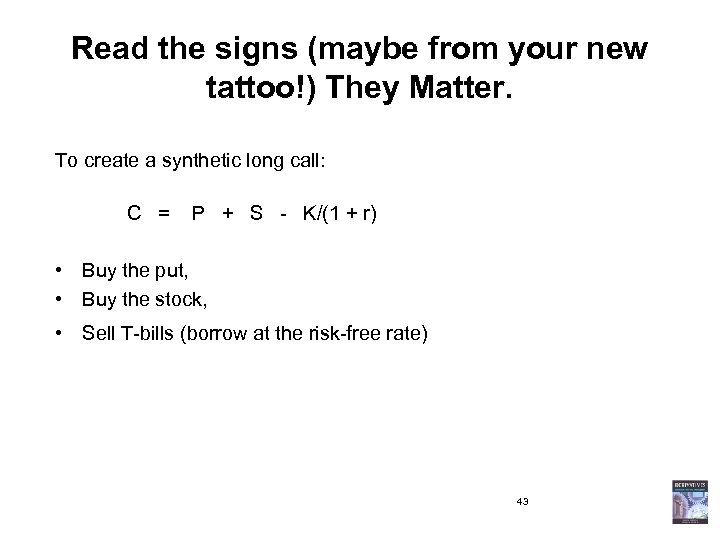Read the signs (maybe from your new tattoo!) They Matter. To create a synthetic long call: C = P + S - K/(1 + r) • Buy the put, • Buy the stock, • Sell T-bills (borrow at the risk-free rate) 43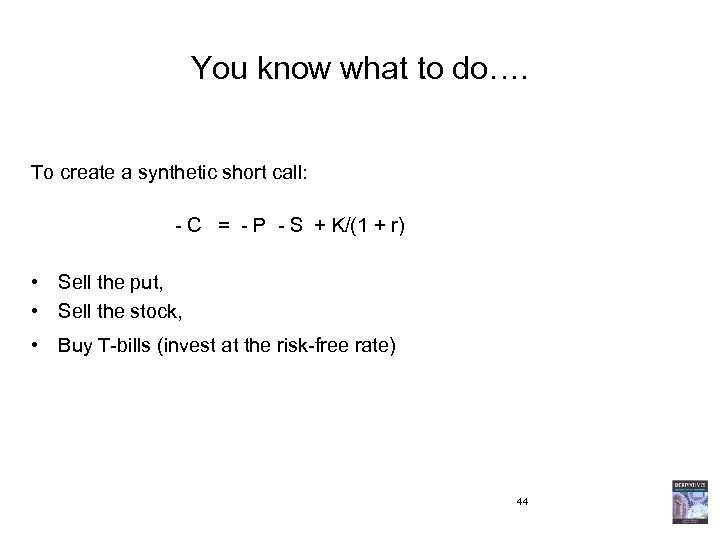You know what to do…. To create a synthetic short call: - C = - P - S + K/(1 + r) • Sell the put, • Sell the stock, • Buy T-bills (invest at the risk-free rate) 44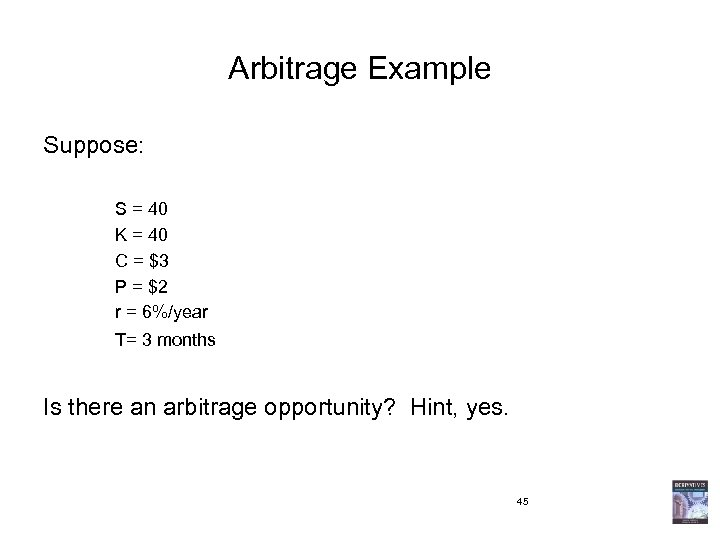Arbitrage Example Suppose: S = 40 K = 40 C = \$3 P = \$2 r = 6%/year T= 3 months Is there an arbitrage opportunity? Hint, yes. 45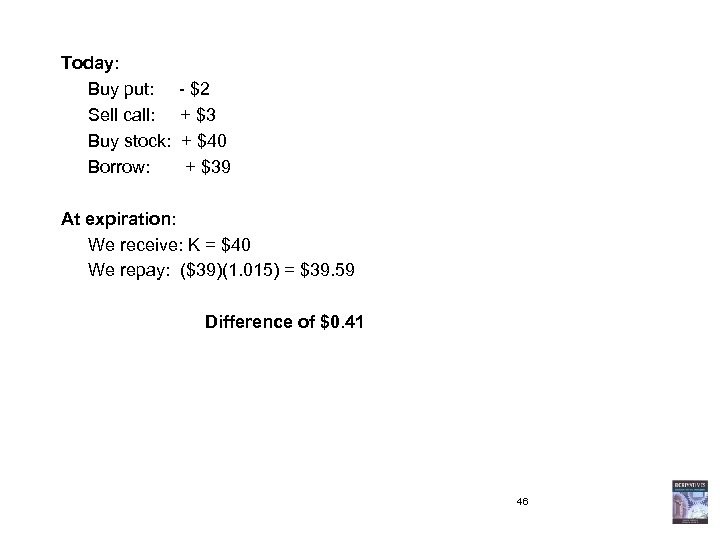Today: Buy put: - \$2 Sell call: + \$3 Buy stock: + \$40 Borrow: + \$39 At expiration: We receive: K = \$40 We repay: (\$39)(1. 015) = \$39. 59 Difference of \$0. 41 46